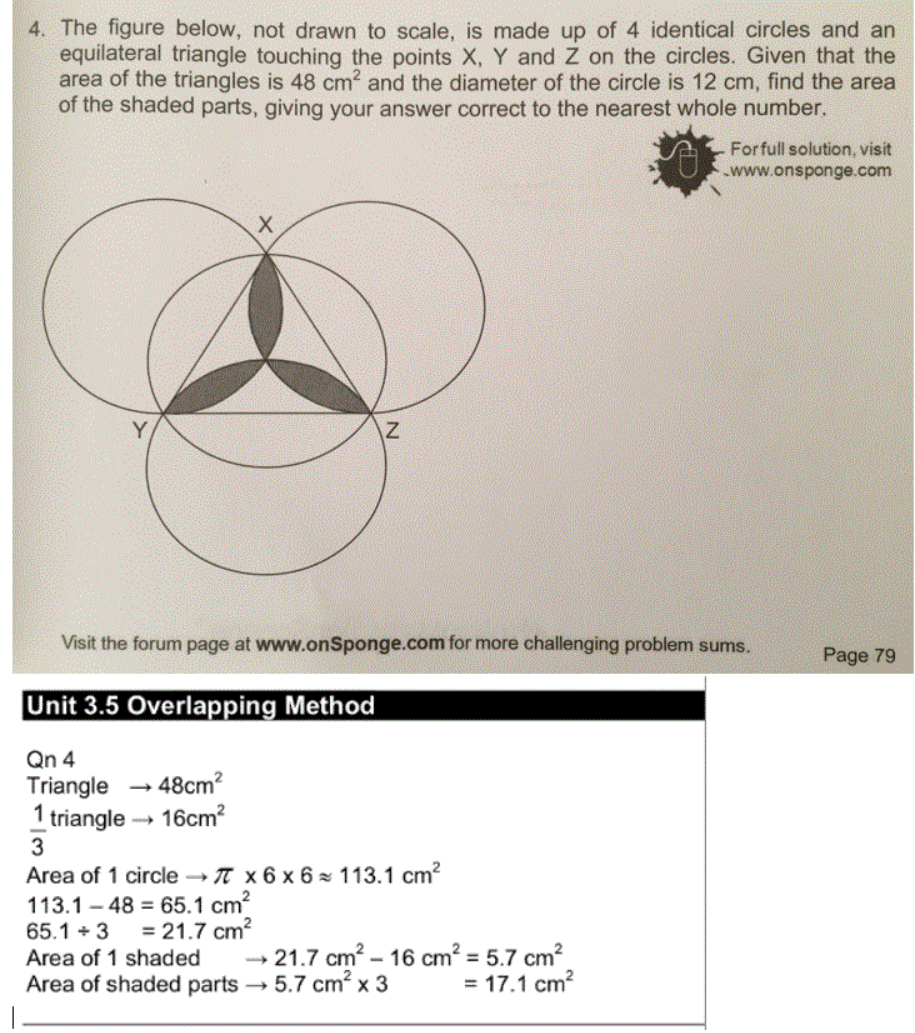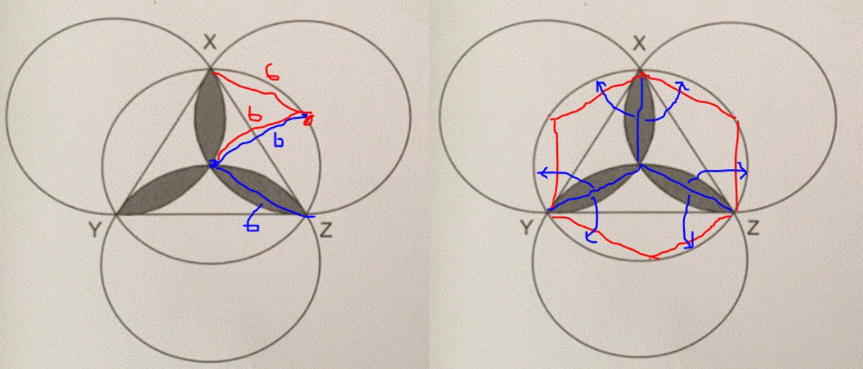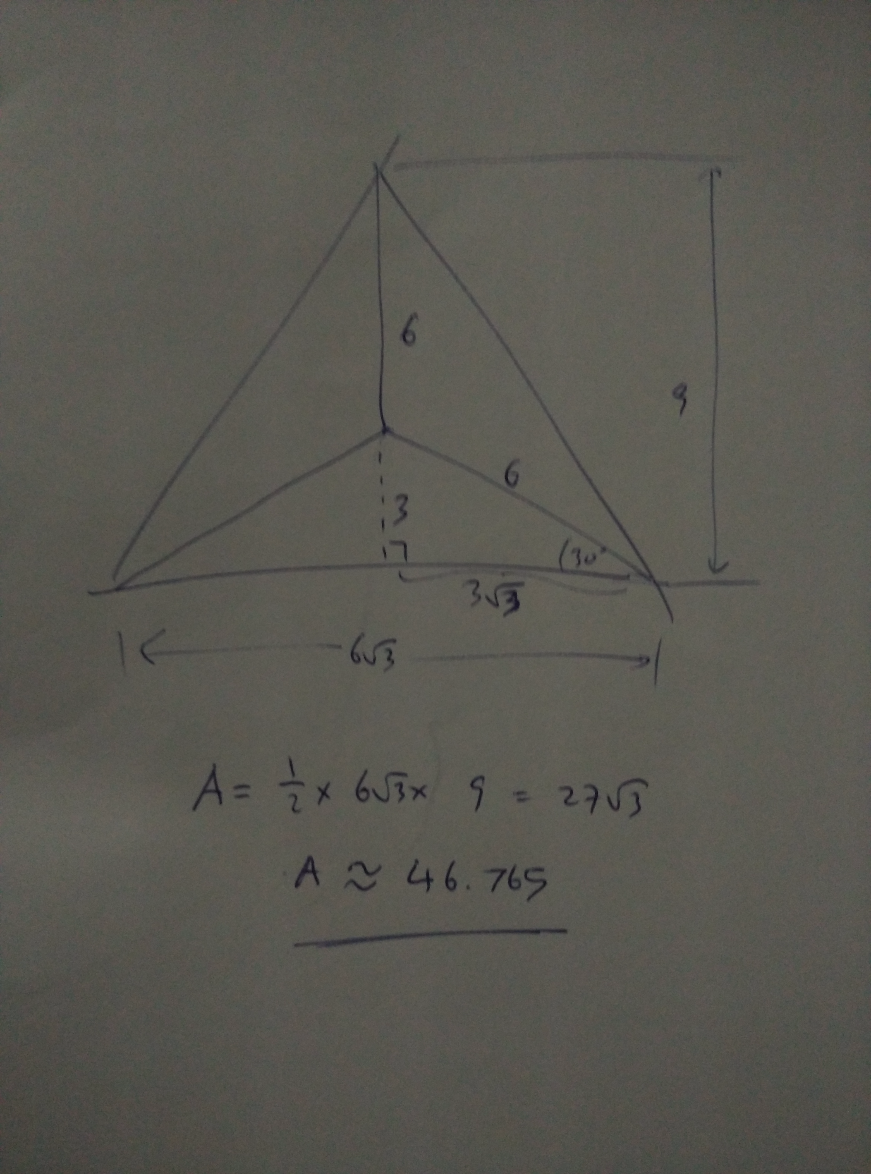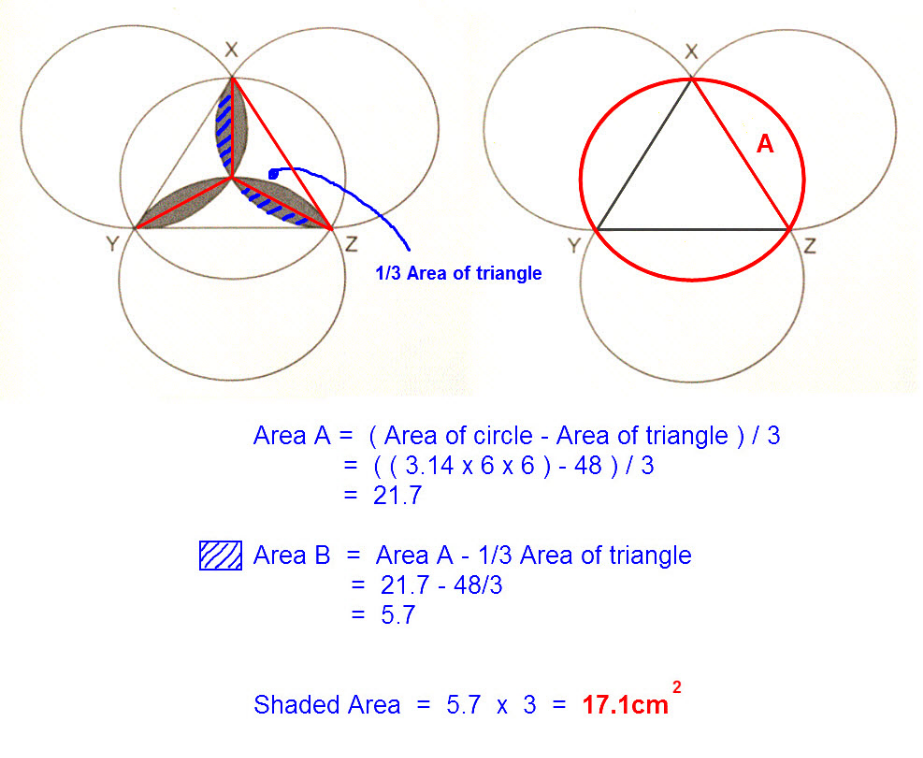# QuestionHi there,

Need help on this circle question. It’s from P6 OnSponge Conquer Problem Sums Unit3.5 Overlapping Method Q4. Have a hard time understanding the solution that would have been much helpful had there been a diagram showing how the answer is arrived at.

Source: AssessmentFrom 1st picture, deduce the 2 chords are the same size, since the circumference of each circle passes the centre of the other identical circle.

Concentrating on the middle circle only:

From 2nd picture, we can flip the “split leaf” into the “edges” of the circle. Remaining is 6 pcs of small triangles or 2 equilateral triangles.

Area of 6 “split leaf” = Area of middle circle -48 -48 = π x 6 x 6 -48 -48

= 17.0 cm2 = 17 cm2 (nearest whole number*)

*for interest only: If I am a killer setter, I will ask like “using π as 3.14, give your answer to the nearest 1 decimal place” (answer will then be 17.0, not 17.1). This can be the 1/2 mark to differentiate the kids.

Hi SAHMom,

This is so refreshing and so creative!

Wait ah, let me spend a bit more time to digest this!

Thanks so much! Really appreciate it.

0 Replies 0 LikesJust wanted to point out that actually the question is not internally consistent, so depending on the method you may get slightly different result. This is because the area of the triangle is not exactly 48 but actually is around 46.765, though this will need a bit of Secondary level math. Hence, if we use just the fact that the radius is 6 cm (which in itself is sufficient), the correct number for the area of the shaded region should be around 19.567.

Granted this is a simplified version to be used for P6 students, but overspecified question like this tends to be a bit dangerous, especially if multiple approaches can be used, which will lead to multiple answers.

Hi Dryu,

Good one to spot that! I totally forgot to check (apologies to Xiao Hu…) and I wholeheartedy agree the “dangerous” part. See a few examples here already. 🙂

0 Replies 0 LikesThis solution steps is similar to the method derived by your OnSponge book.

SAHMom has highlighted another interesting way of looking at the figure which you may want to give it a thought also.